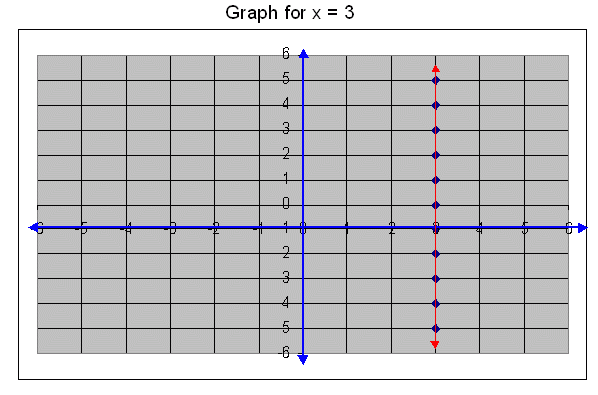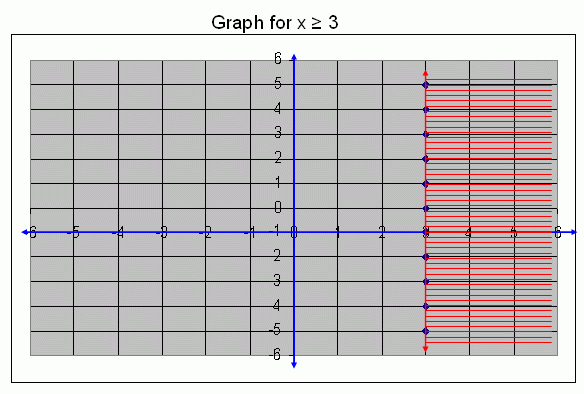Name: ___________________Date:___________________

 Example: Draw a graph for x ³ 3. Solution: First we draw a graph for a straight line x = 3. It means that in this straight line the x coordinate is constant that is 3.x ³ 3 means, that x = 3 or x > 3, all these points are satisfies this condition. Therefore, the graph for x ³ 3 is,Directions: Draw the graphs for the following condition. 1. Draw a graph for x ³ 3. 2. Draw a graph for x ³ 2. 3. Draw a graph for x ³ 6. 4. Draw a graph for x ³ 4. 5. Draw a graph for x ³ 1.